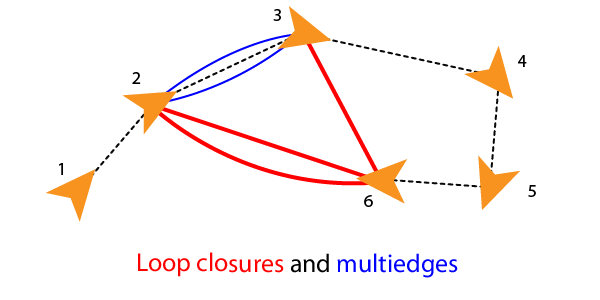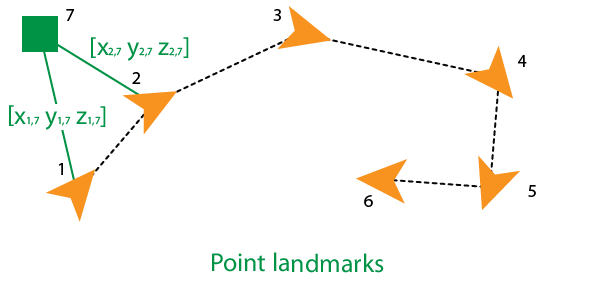poseGraph3D

Create 3-D pose graph

Description

A poseGraph3D object stores information for a 3-D pose graph representation. A pose graph contains nodes connected by edges. Each node estimate is connected to the graph by edge constraints that define the relative pose between nodes and the uncertainty on that measurement.

To construct a pose graph iteratively, use the addRelativePose function to add relative pose estimates and connect them to an existing node with specified edge constraints. Pose nodes must be specified relative to a pose node. Specify the uncertainty of the measurement using an information matrix.Adding an edge between two nonsequential nodes creates a loop closure in the graph. Multiple edges or multiedges between node pairs are also supported, which includes loop closures. To add additional edge constraints or loop closures, specify the node IDs using the addRelativePose function. When optimizing the pose graph, the optimizePoseGraph function finds a solution to satisfy all these edge constraints.To add landmark point nodes, use the addPointLandmark function. This function specifies nodes as xyz-points without orientation estimates. Landmarks must be specified relative to a pose node.For 2-D pose graphs, see poseGraph.

For an example that builds and optimizes a 3-D pose graph from real-world sensor data, see Landmark SLAM Using AprilTag Markers.

Creation

Description

example

poseGraph = poseGraph3D creates a 3-D pose graph object. Add poses using addRelativePose to construct a pose graph iteratively.

poseGraph = poseGraph3D('MaxNumEdges',maxEdges,'MaxNumNodes',maxNodes) specifies an upper bound on the number of edges and nodes allowed in the pose graph when generating code. This limit is only required when generating code.

Properties

expand all

Number of nodes in pose graph, specified as a positive integer. Each node represents a pose measurement or a point landmark measurement. To specify relative poses between nodes, use addRelativePose. To specify a landmark pose, use addLandmarkPose. To get a list of all nodes, use edgeNodePairs.

Number of edges in pose graph, specified as a nonnegative integer. Each edge connects two nodes in the pose graph. Loop closure edges and landmark edges are included.

Number of loop closures in pose graph, specified as a nonnegative integer. To get the edge IDs of the loop closures, use the LoopClosureEdgeIDs property.

Loop closure edges IDs, specified as a vector of edge IDs.

Landmark node IDs, specified as a vector of IDs for each node.

Object Functions

 addPointLandmark Add landmark point node to pose graph addRelativePose Add relative pose to pose graph copy Create copy of pose graph edgeNodePairs Edge node pairs in pose graph edgeConstraints Edge constraints in pose graph edgeResidualErrors Compute pose graph edge residual errors findEdgeID Find edge ID of edge nodeEstimates Poses of nodes in pose graph removeEdges Remove loop closure edges from graph show Plot pose graph

Examples

collapse all

Optimize a pose graph based on the nodes and edge constraints. The pose graph used in this example is taken from the MIT Dataset and was generated using information extracted from a parking garage.

Load the pose graph from the MIT dataset. Inspect the poseGraph3D object to view the number of nodes and loop closures.

disp(pg);
poseGraph3D with properties:

NumNodes: 1661
NumEdges: 6275
NumLoopClosureEdges: 4615
LoopClosureEdgeIDs: [128 129 130 132 133 134 135 137 138 139 140 ... ]
LandmarkNodeIDs: [1x0 double]

Plot the pose graph with IDs off. Red lines indicate loop closures identified in the dataset.

title('Original Pose Graph')
show(pg,'IDs','off');
view(-30,45)Optimize the pose graph. Nodes are adjusted based on the edge constraints and loop closures. Plot the optimized pose graph to see the adjustment of the nodes with loop closures.

updatedPG = optimizePoseGraph(pg);
figure
title('Updated Pose Graph')
show(updatedPG,'IDs','off');
view(-30,45)Carlone, Luca, Roberto Tron, Kostas Daniilidis, and Frank Dellaert. "Initialization Techniques for 3D SLAM: a Survey on Rotation Estimation and its Use in Pose Graph Optimization." 2015 IEEE International Conference on Robotics and Automation (ICRA). 2015, pp. 4597–4604.